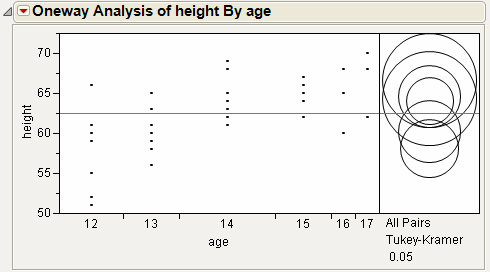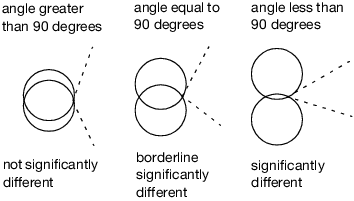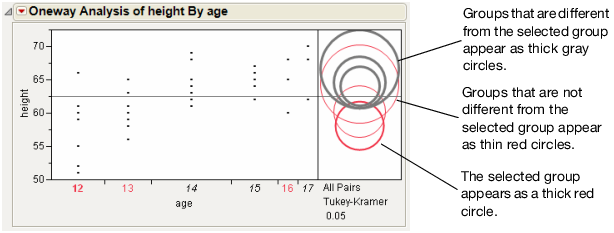Basic Analysis > Oneway Analysis > Compare Means > Using Comparison Circles
Publication date: 11/29/2021

## Using Comparison Circles

Each multiple comparison test, except for the Each Pair Stepwise, Newman-Keuls method, begins with a comparison circles plot, which is a visual representation of group mean comparisons. Figure 6.10 shows the comparison circles for the All Pairs, Tukey HSD method. Other comparison tests lengthen or shorten the radii of the circles.

Note: To permanently hide the comparison circles plot, select File > Preferences > Platforms > Oneway and deselect the Comparison Circles option.

Figure 6.10 Visual Comparison of Group MeansCompare each pair of group means visually by examining the intersection of the comparison circles. The outside angle of intersection tells you whether the group means are significantly different (Figure 6.11).

Circles for means that are significantly different either do not intersect, or intersect slightly, so that the outside angle of intersection is less than 90 degrees.

If the circles intersect by an angle of more than 90 degrees, or if they are nested, the means are not significantly different.

Figure 6.11 Angles of Intersection and SignificanceIf the intersection angle is close to 90 degrees, you can verify whether the means are significantly different by clicking on the comparison circle to select it (Figure 6.12). To deselect circles, click in the white space outside the circles.

Figure 6.12 Highlighting Comparison CirclesRelated Information